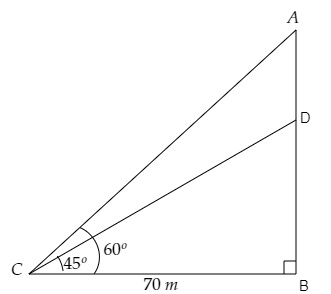# A vertical tower stands on a horizontal plane and is surmounted by a vertical flag-staff. At a point on the plane 70 metres away from the tower, an observer notices that the angles of elevation of the top and the bottom of the flag-staff are respectively $60^{\circ}$ and $45^{\circ}$ Find the height of the flag-staff and that of the tower.

Given:

A vertical tower stands on a horizontal plane and is surmounted by a vertical flag-staff. At a point on the plane 70 metres away from the tower, an observer notices that the angles of elevation of the top and the bottom of the flag-staff are respectively $60^{\circ}$ and $45^{\circ}$.

To do:

We have to find the height of the flag-staff and that of the tower.

Solution:Let $DB$ be the tower and $AD$ be the length of the flag-staff.

Let point $C$ be the point of observation 70 metres away from the tower.

From the figure,

$\mathrm{BC}=70 \mathrm{~m}, \angle \mathrm{DCB}=45^{\circ}, \angle \mathrm{ACB}=60^{\circ}$

Let the height of the tower be $\mathrm{DB}=h \mathrm{~m}$ and the height of the flag-staff be $\mathrm{AD}=x \mathrm{~m}$.

This implies,

$\mathrm{AB}=x+h \mathrm{~m}$

We know that,

$\tan \theta=\frac{\text { Opposite }}{\text { Adjacent }}$

$=\frac{\text { DB }}{BC}$

$\Rightarrow \tan 45^{\circ}=\frac{h}{70}$

$\Rightarrow 1=\frac{h}{70}$

$\Rightarrow h=70(1) \mathrm{~m}$

$\Rightarrow h=70 \mathrm{~m}$

Similarly,

$\tan \theta=\frac{\text { Opposite }}{\text { Adjacent }}$

$=\frac{\text { AB }}{BC}$

$\Rightarrow \tan 60^{\circ}=\frac{x+h}{70}$

$\Rightarrow \sqrt3=\frac{x+70}{70}$

$\Rightarrow x+70=70\sqrt3 \mathrm{~m}$

$\Rightarrow x=70(\sqrt3-1) \mathrm{~m}$

$\Rightarrow x=70(1.732-1) \mathrm{~m}$

$\Rightarrow x=70(0.732) \mathrm{~m}$

$\Rightarrow x=51.24 \mathrm{~m}$

Therefore, the height of the flag-staff is $51.24 \mathrm{~m}$ and the height of the tower is $70 \mathrm{~m}$.

Updated on: 10-Oct-2022

860 Views